# Relative Velocity 1 Relative Velocity Previously we stated

• Slides: 16
Download presentationRelative Velocity 1Relative Velocity Previously we stated that all motion is relative 2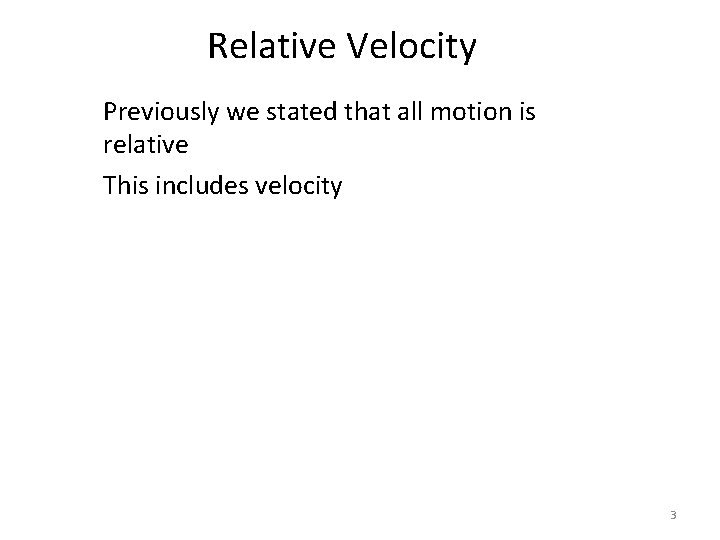Relative Velocity Previously we stated that all motion is relative This includes velocity 3Relative Velocity Previously we stated that all motion is relative This includes velocity The velocity of an object is compared to a reference point. 4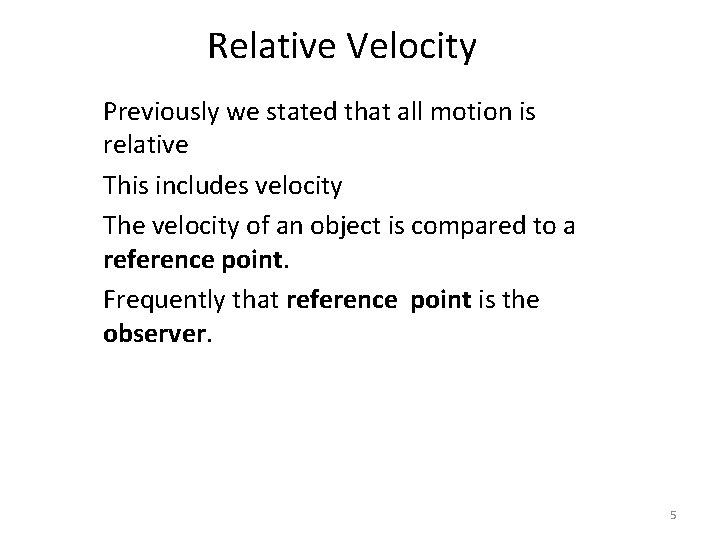Relative Velocity Previously we stated that all motion is relative This includes velocity The velocity of an object is compared to a reference point. Frequently that reference point is the observer. 5The hobo is moving 1. 2 m/s in the boxcar. (relative to the boxcar) The outside observer see the hobo’s motion as 16. 5 m/s relative to the ground. 6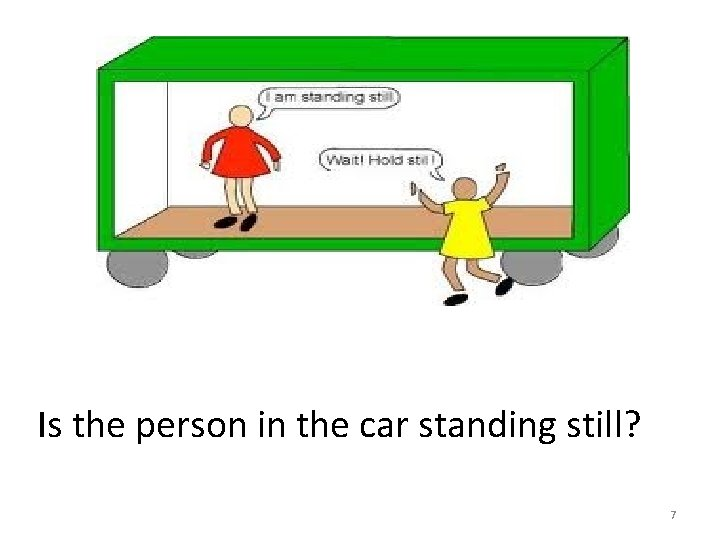Is the person in the car standing still? 7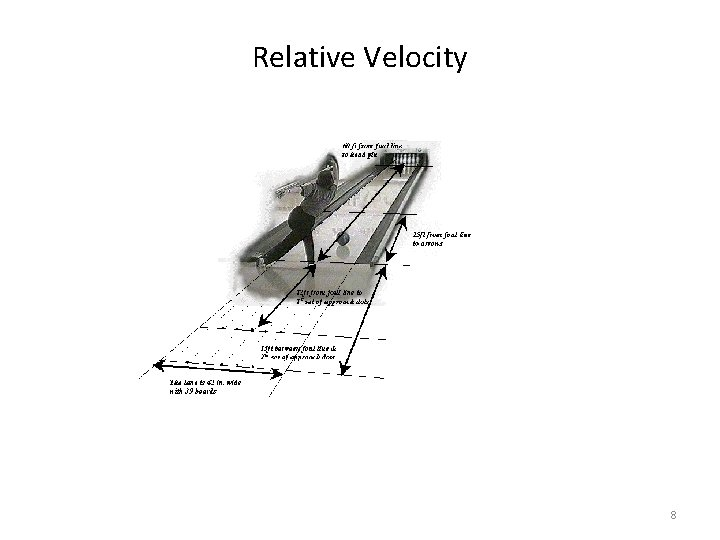Relative Velocity 8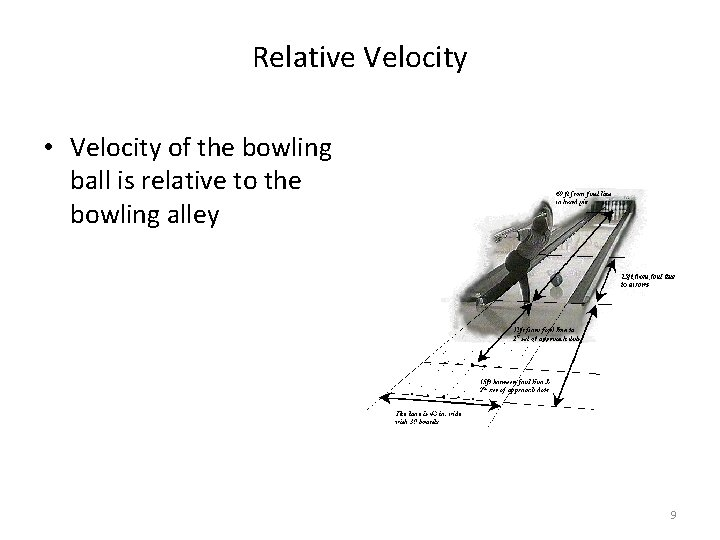Relative Velocity • Velocity of the bowling ball is relative to the bowling alley 9Relative Velocity • Velocity of the bowling ball is relative to the bowling alley • The bowling alley is the frame of reference 10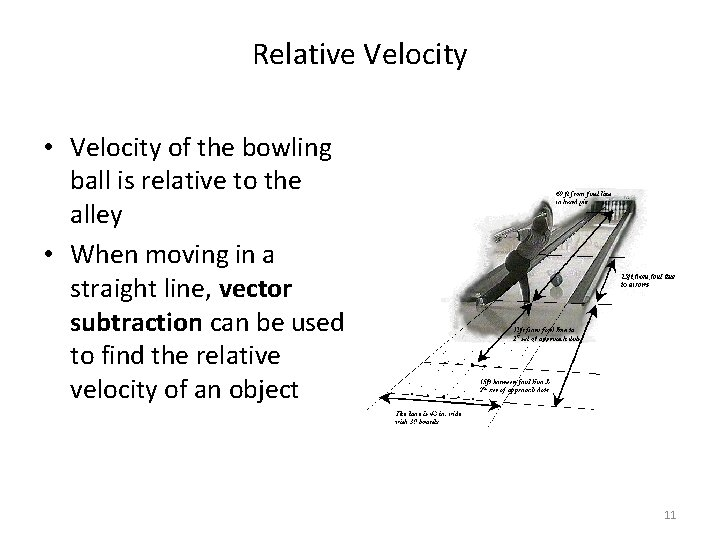Relative Velocity • Velocity of the bowling ball is relative to the alley • When moving in a straight line, vector subtraction can be used to find the relative velocity of an object 11Relative Velocity • B represents the bowling ball 12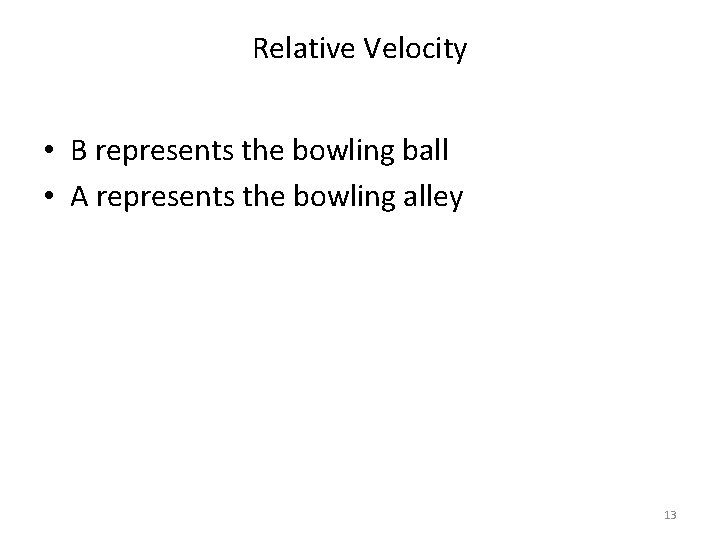Relative Velocity • B represents the bowling ball • A represents the bowling alley 13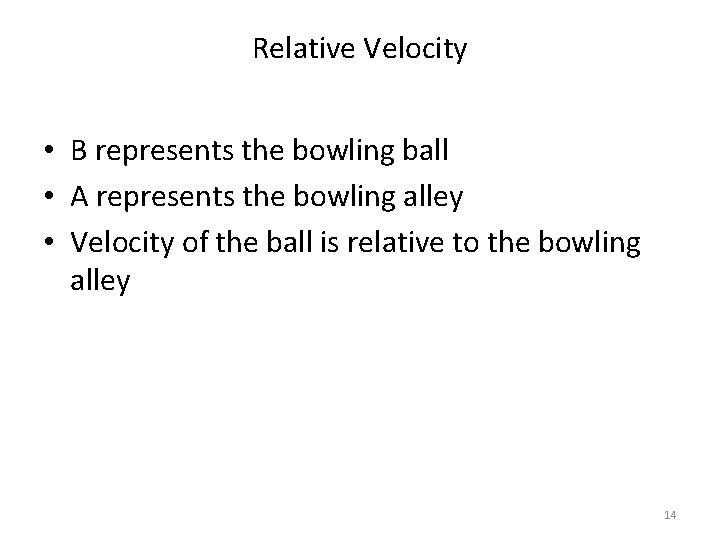Relative Velocity • B represents the bowling ball • A represents the bowling alley • Velocity of the ball is relative to the bowling alley 14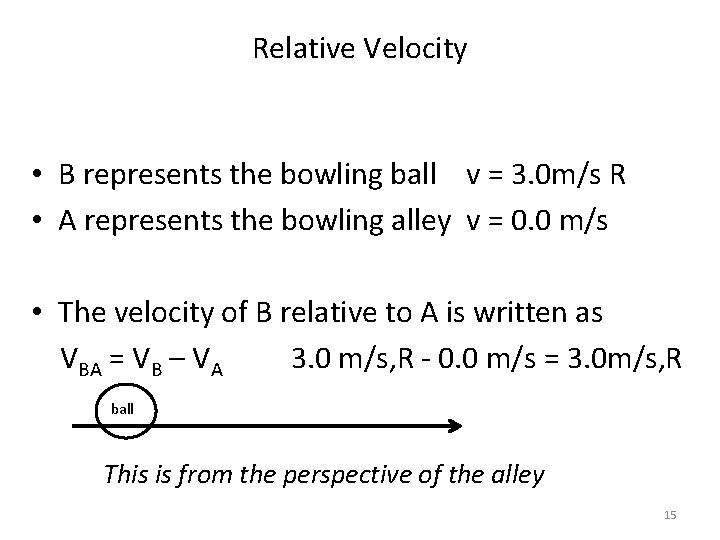Relative Velocity • B represents the bowling ball v = 3. 0 m/s R • A represents the bowling alley v = 0. 0 m/s • The velocity of B relative to A is written as VBA = VB – VA 3. 0 m/s, R - 0. 0 m/s = 3. 0 m/s, R ball This is from the perspective of the alley 15Relative Velocity • B represents the bowling ball v = 3. 0 m/s R • A represents the bowling alley v = 0. 0 m/s • The velocity of A relative to B is written as VAB = VA – VB 0. 0 m/s -3. 0 m/s, R = -3. 0 m/s, R ball This is from the perspective of the ball 16